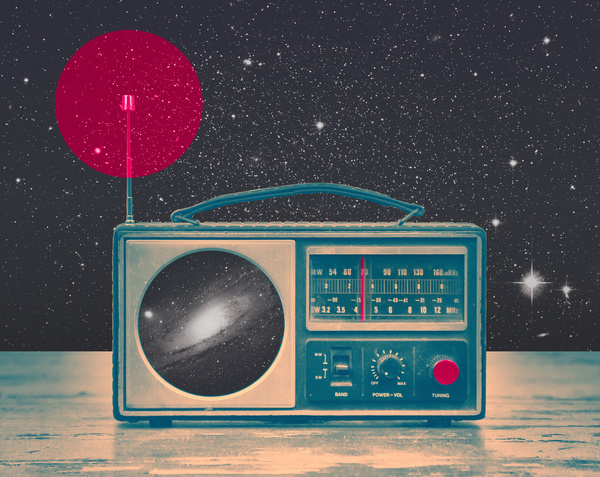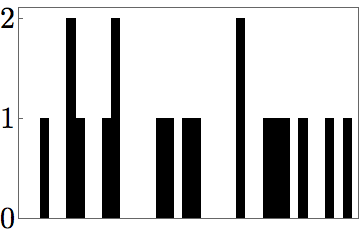# Two states or three?You have a radio that can measure incoming signals from deep space. You measure a signal that appears to be a stream of pulses with relative amplitudes 0, 1, or 2. You measure the signal for a long time and determine that you receive 2s only 15% of the time (i.e. $f(2) = 0.15$). You claim this shows there is some structure to the signals and perhaps you're receiving messages from a distant civilization.

Your partner is more skeptical and insists that what you're measuring is actually the superposition of signals from two identical spinning neutron stars. For simplicity, each neutron star can emit a short burst of radio waves of fixed intensity 1. If both neutron stars emit at the same time, you'll measure a signal of intensity 2.

To prove that this can't be the case, you measure the fraction of 0s, $f(0)$ in the signal and proudly announce that the signal can't possibly be the superposition of noisy neutron stars. What is the greatest possible value you measured for $f(0)$?Assumptions and Details

• You can measure the stream for as long as you like, i.e. you can determine $f(0),\ f(1),\text{ and }f(2)$ to arbitrary accuracy.
• Each pulse lasts for $\tau$ seconds, and the time between pulses is some integer (possibly 0) multiple of $\tau$.
×